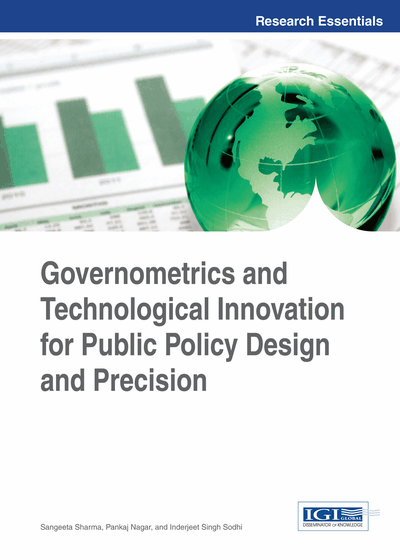Estimation of Agricultural Production

P. C. Gupta (University of Rajasthan – Jaipur, India)
DOI: 10.4018/978-1-4666-5146-3.ch004
Available
\$37.50
No Current Special Offers

Abstract

The successful implementation of e-governance mainly depends on the reliability of its database, as this can be efficiently utilized to give more precise estimates of future production. These predications can be made the basis of the policy for import and export of products like food grain or other crops, which are presently growing in the agricultural fields. Knowing the previous record of the product, as auxiliary variable, one can estimate its future production. This chapter, by constructing practical situational caricatures, emphasizes that the statistical tool like ratio analysis and regression analysis may be helpful in estimating the parameters of the variable in the agriculture field. It includes five illustrations to put forth the idea of application of statistical tools to make effective policy in the field of agriculture.
Chapter Preview
Top

Ratio Method Of Estimation (Ratio Estimator)

Suppose we select a random sample of size n from N units of the population with simple random sampling without replacement and wish to estimate R the population ratio of character Y to that of auxiliary variable X., (1) whereandare population means of characters Y & X respectively. Let the sample observations be (y1, x1), (y2, x2)… (yn, xn) and letandbe the corresponding sampling means. We define the following

(2)

Also their sample estimators

(3)

Let p and r be the population and sample correlation coefficients between Y and X respectively. Then the estimator of R its bias and mean square error to first degree approximation (Sukhatme et. al. 1984) are given as

(4)(5)(6) and the consistent estimators of this bias and M.sc are given as:(7)(8) where, however, have population value, is known

Complete Chapter List

Search this Book:
Reset